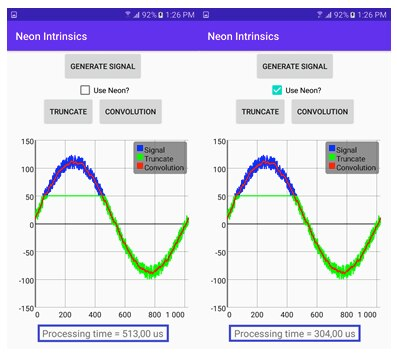## Convolution

Finally, in this section of the guide we look at how to implement a 1D convolution with Neon intrinsics.

Simply speaking, to calculate the convolution, we must have the input signal. Also, you define the kernel. This kernel is typically much shorter than the input signal and varies between applications. Developers use different kernels to smooth or filter noisy signals or to detect edges.

A 2D convolution is also used in convolutional neural networks to find image features.

Our example has a 16-element kernel. In this kernel, each element has the same value of 1. We slide this kernel along the input signal and, at each position, multiply the input element by all kernel values. Then we sum up the resulting products. After normalization, our kernel works as the moving average.

Here is the C++ implementation of this algorithm:

```#define KERNEL_LENGTH 16

// Kernel
int8_t kernel[KERNEL_LENGTH] = {1, 1, 1, 1, 1, 1, 1, 1, 1, 1, 1, 1, 1, 1, 1, 1},

void convolution() {
auto offset = -KERNEL_LENGTH / 2;

// Get kernel sum (for normalization)
auto kernelSum = getSum(kernel, KERNEL_LENGTH);

// Calculate convolution
for (int i = 0; i < SIGNAL_LENGTH; i++) {
int convSum = 0;

for (int j = 0; j < KERNEL_LENGTH; j++) {
convSum += kernel[j] * inputSignal[i + offset + j];
}

inputSignalConvolution[i] = (uint8_t)(convSum / kernelSum);
}
}
```

As you can see, this code uses two for loops: one over the input signal elements and the other over the kernel. To improve performance, we could employ manual loop unrolling. Loop unrolling, also known as loop unwinding, is a loop transformation technique that attempts to optimize a program's execution speed at the expense of its binary size, which is an approach known as space–time tradeoff. The transformation can be undertaken manually by the programmer or by an optimizing compiler. and replace the nested for loop with the hardcoded indexes, las you can see in the following code:

```convSum += kernel * inputSignal[i + offset + 1];
convSum += kernel * inputSignal[i + offset + 2];
…
convSum += kernel * inputSignal[i + offset + 15];
```

However, the kernel length is the same as the number of items that can transfer to the CPU registers. This means that we can employ Neon intrinsics and completely unroll the second loop, as you can see here:

```void convolutionNeon() {
auto offset = -KERNEL_LENGTH / 2;

// Get kernel sum (for normalization)
auto kernelSum = getSum(kernel, KERNEL_LENGTH);

// Load kernel
int8x16_t kernelNeon = vld1q_s8(kernel);

// Buffer for multiplication result
int8_t *mulResult = new int8_t[TRANSFER_SIZE];

// Calculate convolution
for (int i = 0; i < SIGNAL_LENGTH; i++) {
// Load input
int8x16_t inputNeon = vld1q_s8(inputSignal + i + offset);

// Multiply
int8x16_t mulResultNeon = vmulq_s8(inputNeon, kernelNeon);

// Store and accumulate
// On A64 the following lines of code can be replaced by a single instruction
// auto convSum = vaddvq_s8(mulResultNeon)
vst1q_s8(mulResult, mulResultNeon);
auto convSum = getSum(mulResult, TRANSFER_SIZE);

// Store result
inputSignalConvolution[i] = (uint8_t) (convSum / kernelSum);
}

delete mulResult;
}
```

First, we load the data to the CPU registers from the kernel and input signal. Then, we process the data with Neon SIMD, and store the results back to memory. In our example, we store the data in the `inputSignalConvolution` array).

The preceding code is compatible with Arm v7 and newer architectures. However, on AArch64, we can improve the code by using the `vaddvq_s8` function that sums elements across the vector. These are used to sum values under the kernel. See comments in the following code.

To test the code, we use another Java-to-native binding, as you can see here:

```extern "C"
JNIEXPORT jbyteArray JNICALL
Java_com_example_neonintrinsicssamples_MainActivity_convolution(
JNIEnv *env, jobject thiz, jboolean useNeon) {

auto start = now();

#if HAVE_NEON

if(useNeon)
convolutionNeon();
else

#endif

convolution();

processingTime = usElapsedTime(start);

return nativeBufferToByteArray(env, inputSignalConvolution, SIGNAL_LENGTH);
}
```

After rerunning the app using Neon Intrinsics optimizations, the same results are achieved but with only half of the processing time. You can see the processing time in the label at the bottom of the following screenshot: